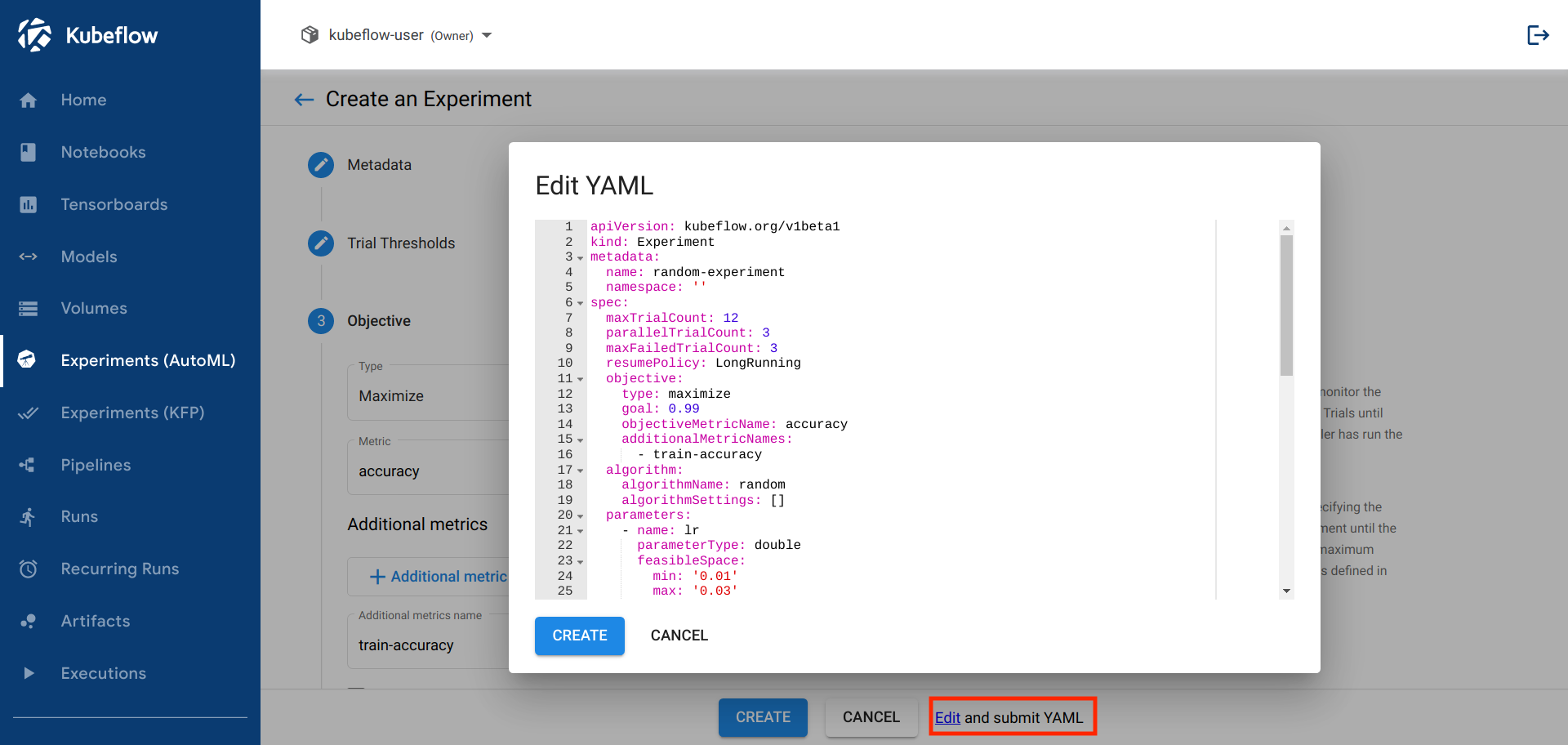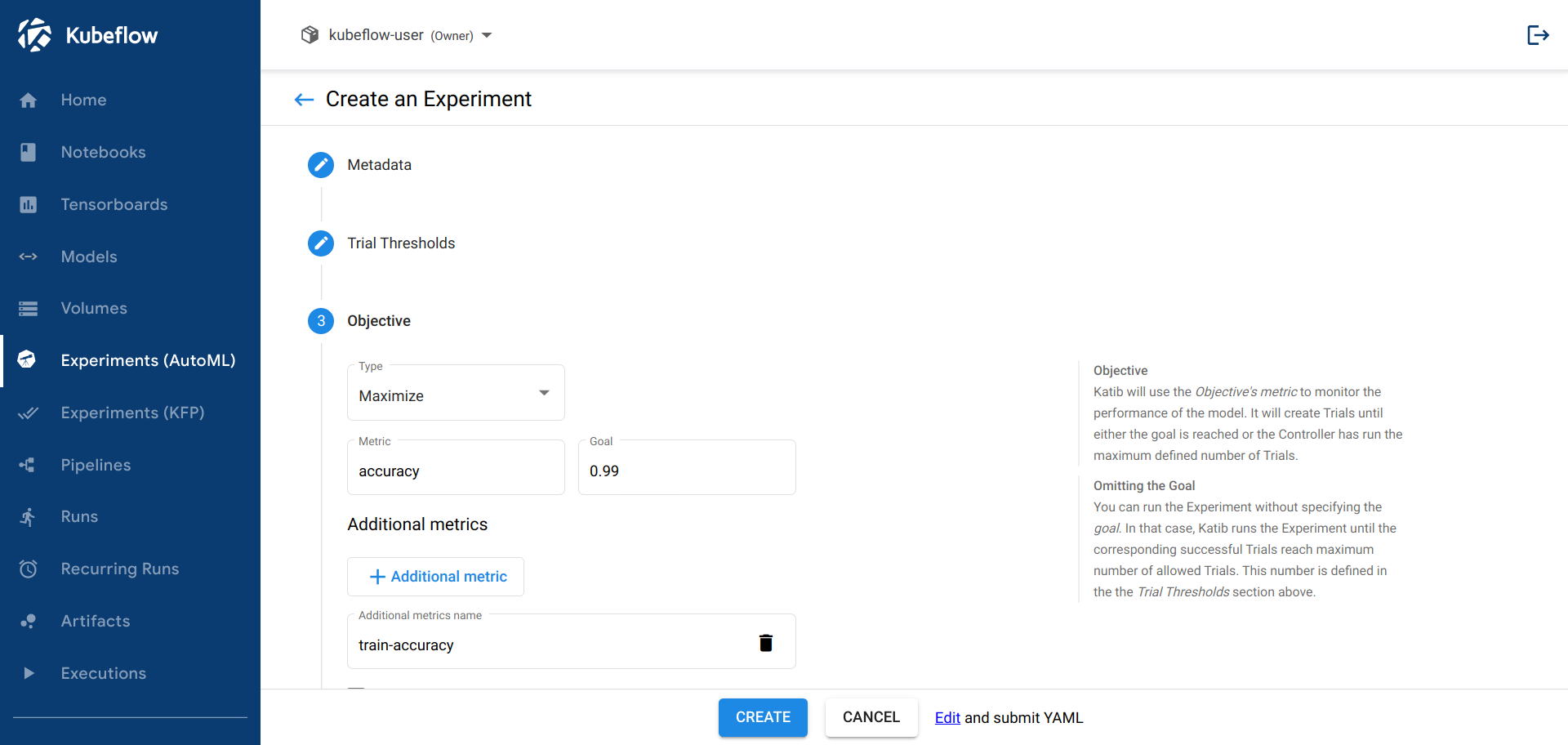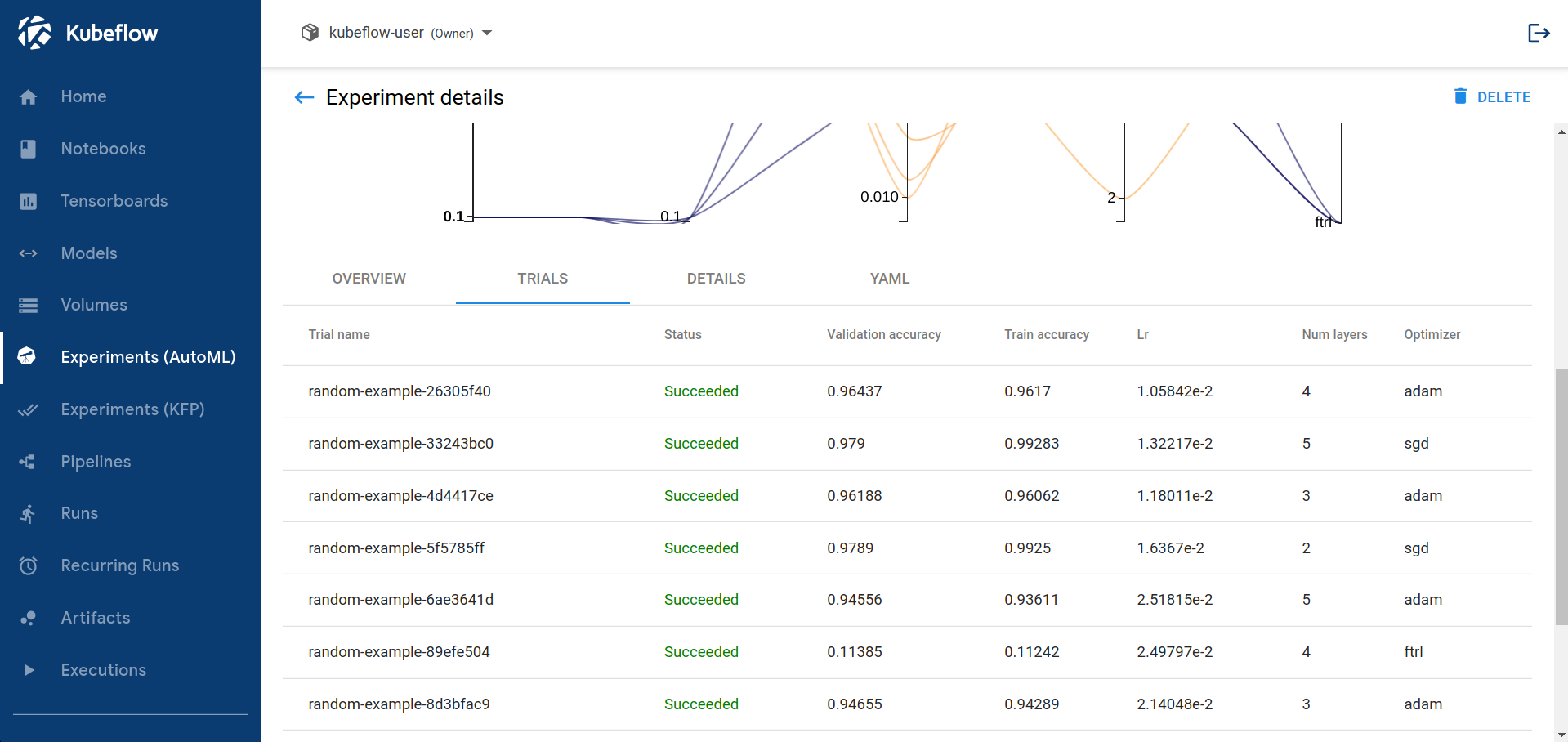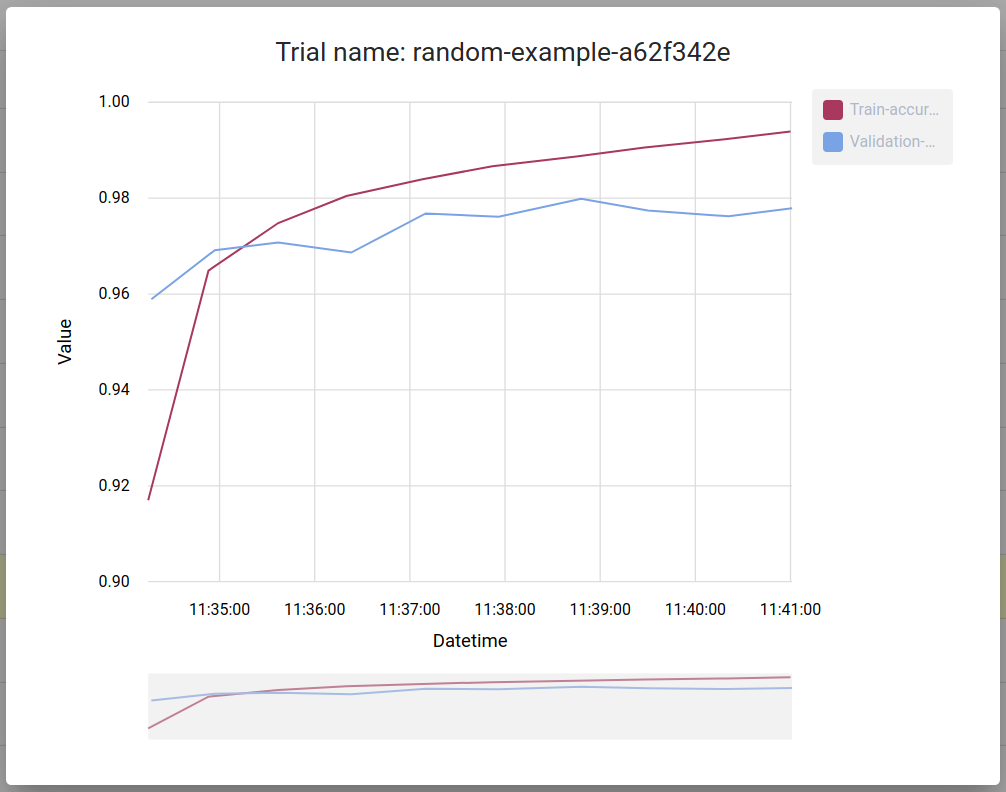# Running an Experiment

How to configure and run a hyperparameter tuning or neural architecture search experiment in Katib

This guide describes how to configure and run a Katib experiment. The experiment can perform hyperparameter tuning or a neural architecture search (NAS) (alpha), depending on the configuration settings.

For an overview of the concepts involved, check the introduction to Katib.

## Packaging your training code in a container image

Katib and Kubeflow are Kubernetes-based systems. To use Katib, you must package your training code in a Docker container image and make the image available in a registry. Check the Docker documentation and the Kubernetes documentation.

## Configuring the experiment

To create a hyperparameter tuning or NAS experiment in Katib, you define the experiment in a YAML configuration file. The YAML file defines the range of potential values (the search space) for the parameters that you want to optimize, the objective metric to use when determining optimal values, the search algorithm to use during optimization, and other configurations.

As a reference, you can use the YAML file of the random search algorithm example.

The list below describes the fields in the YAML file for an experiment. The Katib UI offers the corresponding fields. You can choose to configure and run the experiment from the UI or from the command line.

### Configuration spec

These are the fields in the experiment configuration spec:

• parameters: The range of the hyperparameters or other parameters that you want to tune for your machine learning (ML) model. The parameters define the search space, also known as the feasible set or the solution space. In this section of the spec, you define the name and the distribution (discrete or continuous) of every hyperparameter that you need to search. For example, you may provide a minimum and maximum value or a list of allowed values for each hyperparameter. Katib generates hyperparameter combinations in the range based on the hyperparameter tuning algorithm that you specify. Refer to the `ParameterSpec` type.

• objective: The metric that you want to optimize. The objective metric is also called the target variable. A common metric is the model’s accuracy in the validation pass of the training job (validation-accuracy). You also specify whether you want Katib to maximize or minimize the metric.

Katib uses the `objectiveMetricName` and `additionalMetricNames` to monitor how the hyperparameters work with the model. Katib records the value of the best `objectiveMetricName` metric (maximized or minimized based on `type`) and the corresponding hyperparameter set in the experiment’s `.status.currentOptimalTrial.parameterAssignments`. If the `objectiveMetricName` metric for a set of hyperparameters reaches the `goal`, Katib stops trying more hyperparameter combinations.

You can run the experiment without specifying the `goal`. In that case, Katib runs the experiment until the corresponding successful trials reach `maxTrialCount`. `maxTrialCount` parameter is described below.

The default way to calculate the experiment’s objective is:

• When the objective `type` is `maximize`, Katib compares all maximum metric values.

• When the objective `type` is `minimize`, Katib compares all minimum metric values.

To change the default settings, define `metricStrategies` with various rules (`min`, `max` or `latest`) to extract values for each metric from the experiment’s `objectiveMetricName` and `additionalMetricNames`. The experiment’s objective value is calculated in accordance with the selected strategy.

For example, you can set the parameters in your experiment as follows:

``````. . .
objectiveMetricName: accuracy
type: maximize
metricStrategies:
- name: accuracy
value: latest
. . .
``````

where the Katib controller is searching for the best maximum from the all latest reported `accuracy` metrics for each trial. Check the metrics strategies example. The default strategy type for each metric is equal to the objective `type`.

Refer to the `ObjectiveSpec` type.

• parallelTrialCount: The maximum number of hyperparameter sets that Katib should train in parallel. The default value is 3.

• maxTrialCount: The maximum number of trials to run. This is equivalent to the number of hyperparameter sets that Katib should generate to test the model. If the `maxTrialCount` value is omitted, your experiment will be running until the objective goal is reached or the experiment reaches a maximum number of failed trials.

• maxFailedTrialCount: The maximum number of trials allowed to fail. This is equivalent to the number of failed hyperparameter sets that Katib should test. Katib recognizes trials with a status of `Failed` or `MetricsUnavailable` as `Failed` trials, and if the number of failed trials reaches `maxFailedTrialCount`, Katib stops the experiment with a status of `Failed`.

• algorithm: The search algorithm that you want Katib to use to find the best hyperparameters or neural architecture configuration. Examples include random search, grid search, Bayesian optimization, and more. Check the search algorithm details below.

• trialTemplate: The template that defines the trial. You have to package your ML training code into a Docker image, as described above. `trialTemplate.trialSpec` is your unstructured template with model parameters, which are substituted from `trialTemplate.trialParameters`. For example, your training container can receive hyperparameters as command-line arguments or as environment variables. You have to set the name of your training container in `trialTemplate.primaryContainerName`.

Katib dynamically supports any kind of Kubernetes CRD. In Katib examples, you can find the following job types to train your model:

Refer to the `TrialTemplate` type. Follow the trial template guide to understand how to specify `trialTemplate` parameters, save templates in `ConfigMaps` and support custom Kubernetes resources in Katib.

• metricsCollectorSpec: A specification of how to collect the metrics from each trial, such as the accuracy and loss metrics. Learn the details of the metrics collector below. The default metrics collector is `StdOut`.

• nasConfig: The configuration for a neural architecture search (NAS). Note: NAS is currently in alpha with limited support. You can specify the configurations of the neural network design that you want to optimize, including the number of layers in the network, the types of operations, and more. Refer to the `NasConfig` type.

• graphConfig: The graph config that defines structure for a directed acyclic graph of the neural network. You can specify the number of layers, `input_sizes` for the input layer and `output_sizes` for the output layer. Refer to the `GraphConfig` type.

• operations: The range of operations that you want to tune for your ML model. For each neural network layer the NAS algorithm selects one of the operations to build a neural network. Each operation contains sets of parameters which are described above. Refer to the `Operation` type.

You can find all NAS examples here.

• resumePolicy: The experiment resume policy. Can be one of `LongRunning`, `Never` or `FromVolume`. The default value is `Never`. Refer to the `ResumePolicy` type. To find out how to modify a running experiment and use various restart policies follow the resume an experiment guide.

Background information about Katib’s `Experiment`, `Suggestion` and `Trial` type: In Kubernetes terminology, Katib’s `Experiment` type, `Suggestion` type and `Trial` type is a custom resource (CR). The YAML file that you create for your experiment is the CR specification.

### Search algorithms in detail

Katib currently supports several search algorithms. Refer to the `AlgorithmSpec` type.

Here’s a list of the search algorithms available in Katib:

More algorithms are under development.

You can add an algorithm to Katib yourself. Check the guide to adding a new algorithm and the developer guide.

The algorithm name in Katib is `grid`.

Grid sampling is useful when all variables are discrete (as opposed to continuous) and the number of possibilities is low. A grid search performs an exhaustive combinatorial search over all possibilities, making the search process extremely long even for medium sized problems.

Katib uses the Optuna optimization framework for its grid search.

Setting name Description Example
random_state [int]: Set `random_state` to something other than None for reproducible results. 10

The algorithm name in Katib is `random`.

Random sampling is an alternative to grid search and is used when the number of discrete variables to optimize is large and the time required for each evaluation is long. When all parameters are discrete, random search performs sampling without replacement. Random search is therefore the best algorithm to use when combinatorial exploration is not possible. If the number of continuous variables is high, you should use quasi random sampling instead.

Katib uses the Hyperopt, Goptuna or Optuna optimization framework for its random search.

Katib supports the following algorithm settings:

Setting name Description Example
random_state [int]: Set `random_state` to something other than None for reproducible results. 10

#### Bayesian optimization

The algorithm name in Katib is `bayesianoptimization`.

The Bayesian optimization method uses Gaussian process regression to model the search space. This technique calculates an estimate of the loss function and the uncertainty of that estimate at every point in the search space. The method is suitable when the number of dimensions in the search space is low. Since the method models both the expected loss and the uncertainty, the search algorithm converges in a few steps, making it a good choice when the time to complete the evaluation of a parameter configuration is long.

Katib uses the Scikit-Optimize optimization framework for its Bayesian search. Scikit-Optimize is also known as `skopt`.

Katib supports the following algorithm settings:

Setting Name Description Example
base_estimator [“GP”, “RF”, “ET”, “GBRT” or sklearn regressor, default=“GP”]: Should inherit from `sklearn.base.RegressorMixin`. The `predict` method should have an optional `return_std` argument, which returns `std(Y | x)` along with `E[Y | x]`. If `base_estimator` is one of [“GP”, “RF”, “ET”, “GBRT”], the system uses a default surrogate model of the corresponding type. Learn more information in the skopt documentation. GP
n_initial_points [int, default=10]: Number of evaluations of `func` with initialization points before approximating it with `base_estimator`. Points provided as `x0` count as initialization points. If `len(x0) < n_initial_points`, the system samples additional points at random. Learn more information in the skopt documentation. 10
acq_func [string, default=`"gp_hedge"`]: The function to minimize over the posterior distribution. Learn more information in the skopt documentation. gp_hedge
acq_optimizer [string, “sampling” or “lbfgs”, default=“auto”]: The method to minimize the acquisition function. The system updates the fit model with the optimal value obtained by optimizing `acq_func` with `acq_optimizer`. Learn more information in the skopt documentation. auto
random_state [int]: Set `random_state` to something other than None for reproducible results. 10

#### Hyperband

The algorithm name in Katib is `hyperband`.

Katib supports the Hyperband optimization framework. Instead of using Bayesian optimization to select configurations, Hyperband focuses on early stopping as a strategy for optimizing resource allocation and thus for maximizing the number of configurations that it can evaluate. Hyperband also focuses on the speed of the search.

#### Tree of Parzen Estimators (TPE)

The algorithm name in Katib is `tpe`.

Katib uses the Hyperopt, Goptuna or Optuna optimization framework for its TPE search.

This method provides a forward and reverse gradient-based search.

Katib supports the following algorithm settings:

Setting name Description Example
n_EI_candidates [int]: Number of candidate samples used to calculate the expected improvement. 25
random_state [int]: Set `random_state` to something other than None for reproducible results. 10
gamma [float]: The threshold to split between l(x) and g(x), check equation 2 in this Paper. Value must be in (0, 1) range. 0.25
prior_weight [float]: Smoothing factor for counts, to avoid having 0 probability. Value must be > 0. 1.1

#### Multivariate TPE

The algorithm name in Katib is `multivariate-tpe`.

Katib uses the Optuna optimization framework for its Multivariate TPE search.

Multivariate TPE is improved version of independent (default) TPE. This method finds dependencies among hyperparameters in search space.

Katib supports the following algorithm settings:

Setting name Description Example
n_ei_candidates [int]: Number of Trials used to calculate the expected improvement. 25
random_state [int]: Set `random_state` to something other than None for reproducible results. 10
n_startup_trials [int]: Number of initial Trials for which the random search algorithm generates hyperparameters. 5

#### Covariance Matrix Adaptation Evolution Strategy (CMA-ES)

The algorithm name in Katib is `cmaes`.

Katib uses the Goptuna or Optuna optimization framework for its CMA-ES search.

The Covariance Matrix Adaptation Evolution Strategy is a stochastic derivative-free numerical optimization algorithm for optimization problems in continuous search spaces. You can also use IPOP-CMA-ES and BIPOP-CMA-ES, variant algorithms for restarting optimization when converges to local minimum.

Katib supports the following algorithm settings:

Setting name Description Example
random_state [int]: Set `random_state` to something other than None for reproducible results. 10
sigma [float]: Initial standard deviation of CMA-ES. 0.001
restart_strategy [string, "none", "ipop", or "bipop", default="none"]: Strategy for restarting CMA-ES optimization when converges to a local minimum. "ipop"

#### Sobol’s Quasirandom Sequence

The algorithm name in Katib is `sobol`.

Katib uses the Goptuna optimization framework for its Sobol’s quasirandom search.

The Sobol’s quasirandom sequence is a low-discrepancy sequence. And it is known that Sobol’s quasirandom sequence can provide better uniformity properties.

#### Neural Architecture Search based on ENAS

The algorithm name in Katib is `enas`.

This NAS algorithm is ENAS-based. Currently, it doesn’t support parameter sharing.

Katib supports the following algorithm settings:

Setting Name Type Default value Description
controller_hidden_size int 64 RL controller lstm hidden size. Value must be >= 1.
controller_temperature float 5.0 RL controller temperature for the sampling logits. Value must be > 0. Set value to "None" to disable it in the controller.
controller_tanh_const float 2.25 RL controller tanh constant to prevent premature convergence. Value must be > 0. Set value to "None" to disable it in the controller.
controller_entropy_weight float 1e-5 RL controller weight for entropy applying to reward. Value must be > 0. Set value to "None" to disable it in the controller.
controller_baseline_decay float 0.999 RL controller baseline factor. Value must be > 0 and <= 1.
controller_learning_rate float 5e-5 RL controller learning rate for Adam optimizer. Value must be > 0 and <= 1.
controller_skip_target float 0.4 RL controller probability, which represents the prior belief of a skip connection being formed. Value must be > 0 and <= 1.
controller_skip_weight float 0.8 RL controller weight of skip penalty loss. Value must be > 0. Set value to "None" to disable it in the controller.
controller_train_steps int 50 Number of RL controller training steps after each candidate runs. Value must be >= 1.
controller_log_every_steps int 10 Number of RL controller training steps before logging it. Value must be >= 1.

#### Differentiable Architecture Search (DARTS)

The algorithm name in Katib is `darts`.

Currently, you can’t view results of this algorithm in the Katib UI and you can run experiments only on a single GPU.

Katib supports the following algorithm settings:

Setting Name Type Default value Description
num_epochs int 50 Number of epochs to train model
w_lr float 0.025 Initial learning rate for training model weights. This learning rate annealed down to `w_lr_min` following a cosine schedule without restart.
w_lr_min float 0.001 Minimum learning rate for training model weights.
w_momentum float 0.9 Momentum for training training model weights.
w_weight_decay float 3e-4 Training model weight decay.
w_grad_clip float 5.0 Max norm value for clipping gradient norm of training model weights.
alpha_lr float 3e-4 Initial learning rate for alphas weights.
alpha_weight_decay float 1e-3 Alphas weight decay.
batch_size int 128 Batch size for dataset.
init_channels int 16 Initial number of channels.
print_step int 50 Number of training or validation steps before logging it.
num_nodes int 4 Number of DARTS nodes.
stem_multiplier int 3 Multiplier for initial channels. It is used in the first stem cell.

#### Population Based Training (PBT)

The algorithm name in Katib is `pbt`.

Review the population based training paper for more details about the algorithm.

The PBT service requires a Persistent Volume Claim with RWX access mode to share resources between Suggestion and Trials. Currently, Katib Experiments should have `resumePolicy: FromVolume` to run the PBT algorithm. Learn more about resume policies in this guide.

Katib supports the following algorithm settings:

Setting name Description Example
suggestion_trial_dir The location within the trial container where checkpoints are saved `/var/log/katib/checkpoints/`
n_population Number of trial seeds per generation 40
resample_probability null (default): perturbs the hyperparameter by 0.8 or 1.2. 0-1: resamples the original distribution by the specified probability 0.3
truncation_threshold Exploit threshold for pruning low performing seeds 0.4

### Metrics collector

In the `metricsCollectorSpec` section of the YAML configuration file, you can define how Katib should collect the metrics from each trial, such as the accuracy and loss metrics. Refer to the `MetricsCollectorSpec` type

Your training code can record the metrics into `stdout` or into arbitrary output files. Katib collects the metrics using a sidecar container. A sidecar is a utility container that supports the main container in the Kubernetes Pod.

To define the metrics collector for your experiment:

1. Specify the collector type in the `.collector.kind` field. Katib’s metrics collector supports the following collector types:

• `StdOut`: Katib collects the metrics from the operating system’s default output location (standard output). This is the default metrics collector.

• `File`: Katib collects the metrics from an arbitrary file, which you specify in the `.source.fileSystemPath.path` field. Training container should log metrics to this file in `TEXT` or `JSON` format. If you select `JSON` format, metrics must be line-separated by `epoch` or `step` as follows, and the key for timestamp must be `timestamp`:

``````{"epoch": 0, "foo": “bar", “fizz": “buzz", "timestamp": 1638422847.28721…}
{"epoch": 1, "foo": “bar", “fizz": “buzz", "timestamp": 1638422847.287801…}
{"epoch": 2, "foo": “bar", “fizz": “buzz", "timestamp": "2021-12-02T14:27:50.000035161+09:00"…}
{"epoch": 3, "foo": “bar", “fizz": “buzz", "timestamp": "2021-12-02T14:27:50.000037459+09:00"…}
…
``````

Check the file metrics collector example for `TEXT` and `JSON` format. Also, the default file path is `/var/log/katib/metrics.log`, and the default file format is `TEXT`.

• `TensorFlowEvent`: Katib collects the metrics from a directory path containing a tf.Event. You should specify the path in the `.source.fileSystemPath.path` field. Check the TFJob example. The default directory path is `/var/log/katib/tfevent/`.

• `Custom`: Specify this value if you need to use a custom way to collect metrics. You must define your custom metrics collector container in the `.collector.customCollector` field. Check the custom metrics collector example.

• `None`: Specify this value if you don’t need to use Katib’s metrics collector. For example, your training code may handle the persistent storage of its own metrics.

2. Write code in your training container to print or save to the file metrics in the format specified in the `.source.filter.metricsFormat` field. The default format is `([\w|-]+)\s*=\s*([+-]?\d*(\.\d+)?([Ee][+-]?\d+)?)`. Each element is a regular expression with two subexpressions. The first matched expression is taken as the metric name. The second matched expression is taken as the metric value.

For example, using the default metrics format and `StdOut` metrics collector, if the name of your objective metric is `loss` and the additional metrics are `recall` and `precision`, your training code should print the following output:

``````epoch 1:
loss=3.0e-02
recall=0.5
precision=.4

epoch 2:
loss=1.3e-02
recall=0.55
precision=.5
``````

## Running the experiment

You can run a Katib experiment from the command line or from the Katib UI.

### Running the experiment from the command line

You can use kubectl to launch an experiment from the command line:

``````kubectl apply -f <your-path/your-experiment-config.yaml>
``````

Note:

• If you deployed Katib as part of Kubeflow (your Kubeflow deployment should include Katib), you need to change Kubeflow namespace to your profile namespace.

• (Optional) Katib’s experiments don’t work with Istio sidecar injection. If you are running Kubeflow using Istio, you have to disable sidecar injection. To do that, specify this annotation: `sidecar.istio.io/inject: "false"` in your experiment’s trial template. For examples on how to do it for `Job`, `TFJob` (TensorFlow) or `PyTorchJob` (PyTorch), refer to the getting-started guide.

Run the following command to launch an experiment using the random search algorithm example:

``````kubectl apply -f https://raw.githubusercontent.com/kubeflow/katib/master/examples/v1beta1/hp-tuning/random.yaml
``````

Check the experiment status:

``````kubectl -n kubeflow describe experiment <your-experiment-name>
``````

For example, to check the status of the random search algorithm experiment run:

``````kubectl -n kubeflow describe experiment random
``````

### Running the experiment from the Katib UI

Instead of using the command line, you can submit an experiment from the Katib UI. The following steps assume you want to run a hyperparameter tuning experiment. If you want to run a neural architecture search, access the NAS section of the UI (instead of the HP section) and then follow a similar sequence of steps.

To run a hyperparameter tuning experiment from the Katib UI:

1. Follow the getting-started guide to access the Katib UI.

3. You should be able to view tabs offering you the following options:

• YAML file: Choose this option to supply an entire YAML file containing the configuration for the experiment.• Parameters: Choose this option to enter the configuration values into a form.View the results of the experiment in the Katib UI:

1. You should be able to view the list of experiments:2. Click the name of your experiment. For example, click random-example.

3. There should be a graph showing the level of validation and train accuracy for various combinations of the hyperparameter values (learning rate, number of layers, and optimizer):4. Below the graph is a list of trials that ran within the experiment:5. You can click on trial name to get metrics for the particular trial: